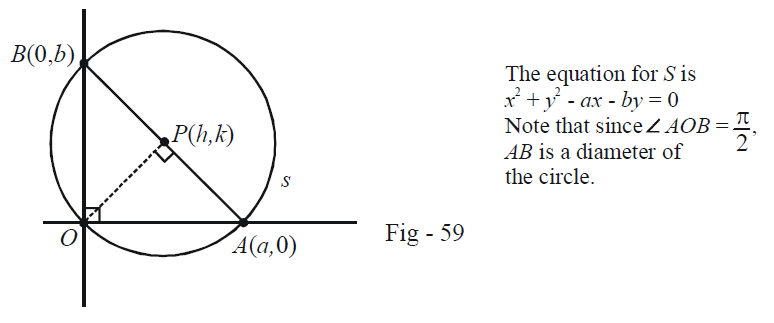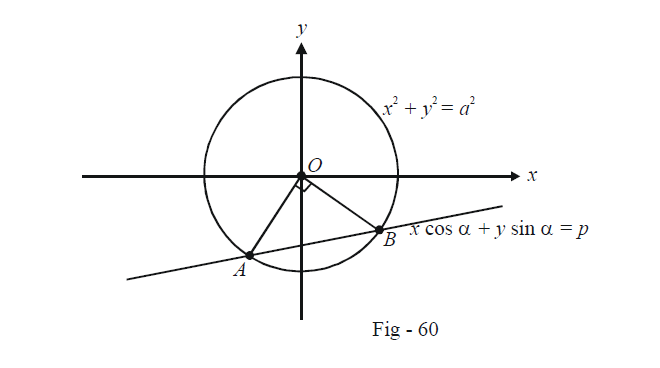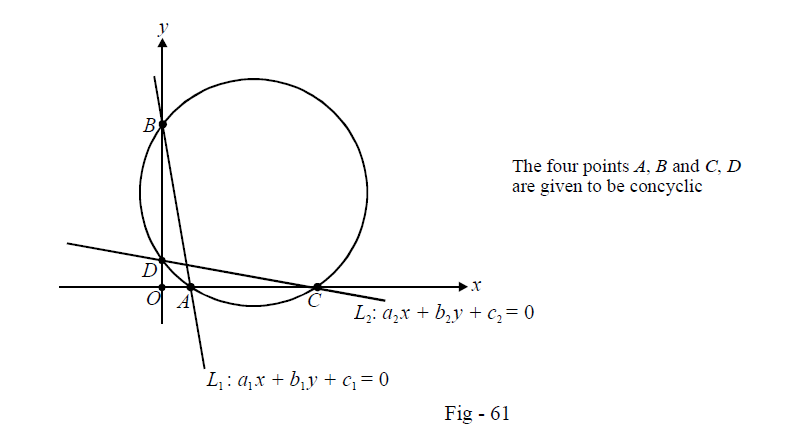# Circles Set-5

Go back to  'SOLVED EXAMPLES'

Learn from the best math teachers and top your exams

• Live one on one classroom and doubt clearing
• Practice worksheets in and after class for conceptual clarity
• Personalized curriculum to keep up with school

Example –13

A circle of radius r passes through the origin O and cuts the axes at A and B. Show that the locus of the foot of the perpendicular from O to AB is

${({x^2} + {y^2})^2}\left( {\frac{1}{{{x^2}}} + \frac{1}{{{y^2}}}} \right) = 4{r^2}$

Solution: Let the co-ordinates of A and B be $$(a,0)$$ and $$(0,b)$$ respectively, so that (like in Example - 8), the equation to the variable circle becomes

${x^2} + {y^2} - ax - by = 0$We have,

${a^2} + {b^2} = 4{r^2}\,\,\,\,\,\,\,\,\,\,\,\,\,\,\,\,\,\,\,\,\,\,\,\,\,\,\,\,\,\,\,\,\,\,\,\,\,\,\,\,\,...(1)$

Let the foot of perpendicular P have the co-ordinates $$(h,k).$$ Since $$OP \bot AB,$$ we obtain

\begin{align}&\,\,\,\,\,\,\,\,\,\,\,\,\,\,\,\,\,\frac{k}{h} \times \frac{b}{{ - a}} = - 1\,.\\&\Rightarrow \quad \frac{k}{a} = \frac{h}{b} = \frac{{\sqrt {{h^2} + {k^2}} }}{{\sqrt {{a^2} + {b^2}} }} = \frac{{\sqrt {{h^2} + {k^2}} }}{{2r}}\\&\Rightarrow \quad a = \frac{{2rk}}{{\sqrt {{h^2} + {k^2}} }},\,\,b = \frac{{2rh}}{{\sqrt {{h^2} + {k^2}} }}\,\,\,\,\,\,\,\,\,\,\,\,\,\,\,\,\,\,\,\,\,\,\,\,\,\,\,\,\,\,\,\,\,\,\,\,\,\,\,\,\,\,\,\,...(2)\end{align}

Using (2) in (1) and $$(x,y)$$ instead of $$(h,k),$$ we obtain the required locus.

Example –14

Find the condition so that the chord $$x\cos \alpha + y\sin \alpha = p$$ subtends a right angle at the centre of the circle $${x^2} + {y^2} = {a^2}$$.

Solution:We can obtain the joint equation J to the pair of lines OA and OB by homogenizing the equation of the circle using the equation of the chord AB.

$J:{x^2} + {y^2} = {a^2}{\left( {\frac{{x\cos \alpha + y\sin \alpha }}{p}} \right)^2}\,\,\,\,\,\,\,\,\,\,\,\,\,\,\,\,\,\,\,\,\,\,\,\,\,\,\,\,\,\,\,\,\,\,\,\,...(1)$

For J to represent two perpendicular lines, we must have in (1),

${\rm{Coeff}}{\rm{. of }}\;{x^2} + {\rm{Coeff.}}\;{\rm{of }}\;{y^2} = 0$

which upon simplification yields the required condition as

${a^2} = 2{p^2}$

Example –15

Suppose that the lines $${a_1}x + {b_1}y + {c_1} = 0$$ and $${a_2}x + {b_2}y + {c_2} = 0$$ intersect the co-ordinate axes at points $$A,\,\,B\,\,$$ and $$C,\,\,D$$ respectively. Find the condition that must be satisfied if these four points are to be concyclic.

Solution: The co-ordinate of $$A,\,\,B\,\,$$ and  $$C,\,\,D$$ can be evaluated to be \begin{align}\left( { - \frac{{{c_1}}}{{{a_1}}},0} \right),\,\,\left( {0, - \frac{{{c_1}}}{{{b_1}}}} \right),\,\,\left( { - \frac{{{c_2}}}{{{a_2}}},0} \right)\end{align} and \begin{align}\left( {0, - \frac{{{c_2}}}{{{b_2}}}} \right).\end{align}Instead of resorting to a detailed calculation, we simply use the result on tangents and secants that we’ve already derived earlier:

$$OA \cdot OC = OD \cdot OB = {l^2}$$

where $$l$$ is the length of the tangent drawn from O to the circle. This gives

\begin{align}&\,\,\,\,\,\,\,\,\,\,\,\,\,\left( { - \frac{{{c_1}}}{{{a_1}}}} \right) \cdot \left( { - \frac{{{c_2}}}{{{a_2}}}} \right) = \left( { - \frac{{{c_2}}}{{{b_2}}}} \right) \cdot \left( { - \frac{{{c_1}}}{{{b_1}}}} \right)\\&\Rightarrow\quad {a_1}{a_2} - {b_1}{b_2} = 0\end{align}

This is the required condition!

Learn from the best math teachers and top your exams

• Live one on one classroom and doubt clearing
• Practice worksheets in and after class for conceptual clarity
• Personalized curriculum to keep up with school

0